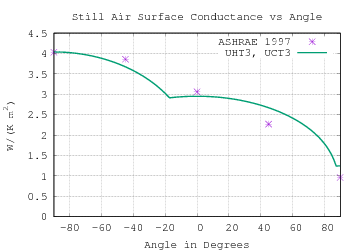# Voluntocracy

Governance by those who do the work.

## Sunday, June 12, 2011

### Free Convection from Rectangular Plate

Have completed a milestone in theory of convection  (corrected 2012-01-08)Free convection correlations for inclined rectangular plates involve characteristic-lengths LHLW, and L* (area divided by perimeter). Each correlation is computed using its own characteristic-length. Flows are then compared and selected as convective surface-conductances (h).

Proposed is the complete free convective surface conductance for one side of a flat LH by LW rectangular isothermal plate inclined θ around the level edge which is LW long.

 L* = LH⋅LW / (2 (LH+LW)) R = min(LH, LW)/2 Pr = cp⋅μ/k Ra(L) = |ΔT|⋅g⋅ρ2⋅cp⋅L3 / (T⋅μ⋅k) HHT*(Ra*) = {0.65 + 0.36 Ra*1/6}2 1≤Ra*≤1.5×109 θ=−90° T9.3(Ra) = {.825 + .387 Ra1/6 [1+(.492/Pr)9/16]8/27 }2 1≤Ra≤1012 θ=0° Nu45(Ra) = 0.544 Ra1/5 [1+(0.785/Pr)3/5]1/3 θ=+90°

 h = k⋅max( Nu45(Ra(R)) R , T9.3(Ra(LH) cos θ) LH ) 0°≤θ≤90° UCT3 h = k⋅max( HHT*(Ra(L*) sin(−θ)) L* , T9.3(Ra(LH) cos θ) LH ) −90°≤θ≤0° UHT3